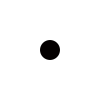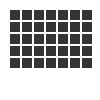# Utility¶

`var`(name, type, default=None, min=0, max=255, value=None, step=None, steps=256.0)

Creates a live variable, which can be manipulated using the variables UI, socket server or live coding shell.

The first two arguments are the variable name and type (NUMBER, TEXT, BOOLEAN or BUTTON).

An optional third argument is the default (initial) value. For NUMBER variables, the minimum and maximum values for the variable can be indicated.

Finally, there are two options for setting the step length on the variables interface. This is the “jump” between values if you don’t want to use a continuous scale. You can either set a fixed number of steps using the `steps` option, or set a step length with the `step` option.

`random`(v1=None, v2=None)

Returns a random number that can be assigned to a variable or a parameter. When no parameters are supplied, returns a floating-point (decimal) number between 0.0 and 1.0 (including 0.0 and 1.0). When one parameter is supplied, returns a number between 0 and this parameter. When two parameters are supplied, returns a number between the first and the second parameter.```r = random() # returns a float between 0 and 1
r = random(2.5) # returns a float between 0 and 2.5
r = random(-1.0, 1.0) # returns a float between -1.0 and 1.0
r = random(5) # returns an int between 0 and 5
r = random(1, 10) # returns an int between 1 and 10

# sets the fill to anything from
# black (0.0,0,0) to red (1.0,0,0)
fill(random(), 0, 0)
circle(40, 40, 20)

# Note: new random values are returned each time the script runs.
# The variation can be locked by supplying a custom random seed:

from random import seed
seed(0)
```
`grid`(cols, rows, colSize=1, rowSize=1, shuffled=False)

This command returns an iterable object which can be traversed in a loop.

The first two parameters define the number of columns and rows in the grid. The next two parameters are optional, and set the width and height of one cell in the grid. In each loop iteration, the offset for the current column and row is returned.

If `shuffled` is True, the cells will be returned in a random order.```translate(10, 10)
for x, y in grid(7, 5, 12, 12):
rect(x, y, 10, 10)
```
`fontnames`()

Returns a list of system font faces, in the same format that `font()` expects.

`files`(path='*')

Retrieves all files from a given path and returns their names as a list. Wildcards can be used to specify which files to pick, e.g. ```f = files('*.gif')```

`autotext`(sourceFile)

Accepts a source file name, and generates mock philosophy based on a context-free grammar.